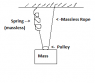# Oscillation of a spring - pulley system

• ineedhelp1234
In summary, a massless spring with a constant of k=196 N/m is used to suspend a mass of M=1.45 kg (including the pulley). The frequency of simple harmonic motion in this system is 2.62 Hz, calculated using the equation f = (1/2π)√(k/m). The rope and pulley arrangement effectively doubles the force felt by the mass, making the system behave as though there are two springs with the same k value.

#### ineedhelp1234

A massless spring of constant k=196 N/m is used to suspend the mass M=1.45 kg (including the pulley), as shown in the attached figure. What is the frequency of simple harmonic motion?

Relevant equations
f = 1/period
T = 2pi*sqrt(M/k)
Tension = 1/2Mg

What I tried...
T(tension on spring) = 1/2Mg <-- since each rope has equal tension?? with that, I found that the mass acting on the spring would be M/2 since the tension is half of the weight?? I feel like this may be where I'm going wrong..

f = 1/(period) = (1/2pi)*sqrt(k/m)
f = 2.62 /hz

I know I'm doing something wrong, I'm just not sure how what I need to change. Any explanations would be great!

#### Attachments

•pulley -spring question physics.png
1.8 KB · Views: 1,135
The tension in the rope is going to vary as the mass moves up and down (this should be obvious, as the spring will change length and so must "administer" a force -kΔx accordingly).

Something to consider is the fact that, thanks to the rope and pulley, the other side of the rope (without the spring) is always going to have the same tension as the side with the spring. This assumes a massless pulley without friction or rotational inertia of course.

Since the tension versus displacement is the same for both ropes, the system behaves as though BOTH ropes have a spring in them! So what is the effective spring constant for two springs in parallel?

Okay that makes a lot of sense :) Would the k of the spring then equal the k of the rope? So k(eff) = 2k ?

ineedhelp1234 said:
Okay that makes a lot of sense :) Would the k of the spring then equal the k of the rope? So k(eff) = 2k ?

Strictly speaking the ideal massless rope doesn't have a spring constant, but I understand what you meanYes, the system behaves as though the "solid" rope were another spring of the same k.

In reality, that rope and pulley arrangement doubles the force that the mass feels from the spring for a given Δx of the spring.